## 1.9  Abstraction of Path Functions

An essential step in the derivation of the local-tuple transformation function C from the coordinate transformation F was the deduction of the relationship between the velocities in the two coordinate systems. We did this by inserting coordinate paths into the coordinate transformation function F, differentiating, and then generalizing the results on the path to arbitrary velocities at a moment. The last step is an example of a more general problem of abstracting a local-tuple function from a path function. Given a function f of a local tuple, a corresponding path-dependent function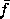[q] is[q] = f o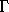[q]. Given, how can we reconstitute f? The local-tuple function f depends on only a finite number of components of the local tuple, anddepends only on the corresponding local components of the path. Sohas the same value for all paths that have that number of components of the local tuple in common. Givenwe can reconstitute f by taking the argument of f, which is a finite initial segment of a local tuple, constructing a path that has this local description, and finding the value offor this path.

Two paths that have the same local description up to the nth derivative are said to osculate with order-n contact. For example, a path and the truncated power series representation of the path up to order n have order-n contact; if fewer than n derivatives are needed by a local-tuple function, the path and the truncated power series representation are equivalent. Let O be a function that generates an osculating path with the given local-tuple components. So O(t, q, v, ...)(t) = q, D(O(t, q, v, ...))(t) = v, and in general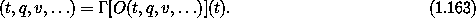The number of components of the local tuple that are required is finite, but unspecified. One way of constructing O is through the truncated power series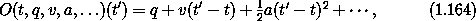where the number of terms is the same as the number of components of the local tuple that are specified.

Given the path functionwe reconstitute the f function as follows. We take the argument of f and construct an osculating path with this local description. Then the value of f is the value offor this osculating path: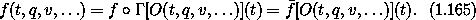Let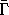be the function that takes a path function and returns the corresponding local-tuple function: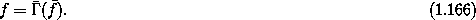From equation (1.165) we see that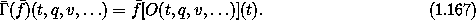The procedure Gamma-bar implements the functionthat reconstitutes a path-dependent function into a local-tuple function:

(define ((Gamma-bar f-bar) local)
((f-bar (osculating-path local)) (time local)))

The procedure osculating-path takes a number of local components and returns a path with these components; it is implemented as a power series.

We can use Gamma-bar to construct the procedure F->C that takes a coordinate transformation F and generates the procedure that transforms local tuples. The procedure F->C constructs a path-dependent procedure f-bar that takes a coordinate path in the primed system and returns the local tuple of the corresponding path in the unprimed coordinate system. It then uses Gamma-bar to abstract f-bar to arbitrary local tuples in the primed coordinate system.

(define (F->C F)
(define (f-bar q-prime)
(define q
(compose F (Gamma q-prime)))
(Gamma q))
(Gamma-bar f-bar))
(show-expression
((F->C p->r)
(->local 't (up 'r 'theta) (up 'rdot 'thetadot))))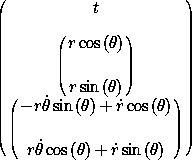Notice that in this definition of F->C we do not explicitly calculate any derivatives. The calculation that led up to the state transformation (1.74) is not needed.

We can also useto make an elegant formula for computing the total time derivative Dt F of the function F: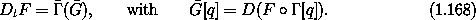The total time derivative can be expressed as a program:

(define (Dt F)
(define (G-bar q)
(D (compose F (Gamma q))))
(Gamma-bar G-bar))

Given a procedure F implementing a local-tuple function and a path q, we construct a new procedure (compose F (Gamma q)). The procedure G-bar implements the derivative of this function of time. We then abstract this off the path with Gamma-bar to give the total time derivative.

Exercise 1.31.  Velocity transformation
Use the procedure Gamma-bar to construct a procedure that transforms velocities given a coordinate transformation. Apply this procedure to the procedure p->r to deduce (again) equation (1.65).

Exercise 1.32.  Path functions and state functions
The local-tuple function f is the same as the local-tuple function() where[q] = f o[q]. On the other hand, the path function[q] and the path function() o[q] are not necessarily the same. Explain. Give examples where they are the same and where they are not the same. Write programs to illustrate the behavior.

#### Lagrange equations at a moment

Given a Lagrangian, the Lagrange equations test paths to determine whether they are realizable paths of the system. The Lagrange equations relate the path and its derivatives. The fact that the Lagrange equations must be satisfied at each moment suggests that we can abstract the Lagrange equations off the path and write them as relations among the local-tuple components of realizable paths.

Let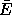[L] be the path-dependent function that produces the residuals of the Lagrange equations (1.18) for the Lagrangian L: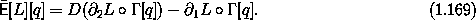Realizable paths q satisfy the Lagrange equations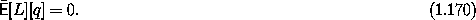The path-dependent Lagrange equations can be converted to local Lagrange equations using: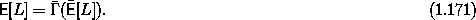The operator E is called the Euler-Lagrange operator. In terms of this operator the Lagrange equations areApplying the definition (1.167) ofyields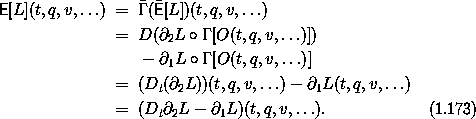So the Euler-Lagrange operator is explicitly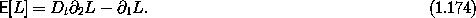The procedure Euler-Lagrange-operator implements E:

(define (Euler-Lagrange-operator L)
(- (Dt ((partial 2) L)) ((partial 1) L))) .

For example, applied to the Lagrangian for the harmonic oscillator, we have

(print-expression
((Euler-Lagrange-operator
(L-harmonic 'm 'k))
(->local 't 'x 'v 'a)))
(+ (* a m) (* k x))

Notice that the components of the local tuple are individually specified. Using equation (1.172), the Lagrange equations for the harmonic oscillator are87

(print-expression
((compose
(Euler-Lagrange-operator (L-harmonic 'm 'k))
(Gamma (literal-function 'x) 4))
't))
(+ (* k (x t)) (* m (((expt D 2) x) t)))

Exercise 1.33.  Properties of E
Let F and G be two Lagrangian-like functions of a local tuple, C be a local-tuple transformation function, and c a constant. Demonstrate the following properties:

a.   E[F + G] = E[F] + E[G]

b.   E[c F] = c E[F]

c.   E[F G] = E[F] G + F E[G] + (Dt F)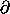2 G +2 F (Dt G)

d.   E[F o C] = Dt (DF o C)2 C + DF o C E[C]

87 Notice that Gamma has one more argument than it usually has. This argument gives the length of the initial segment of the local tuple needed. The default length is 3, giving components of the local tuple up to and including the velocities.